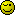# Check form value against database values

That last one owrked perfectly, so as an extension on that I thought I would build into it a way for the user to see what the last contract in the database is of the type they entered, and then eventually to add one to it and ask them if they want to use that one instead. So have put this together, and wondered if you could cast your eye over it and see if you can see anything thats wrong.

``````
\$rightNumber=\$_GET["NumberRight"];
\$contractLast = substr(\$rightNumber, 0, 3);
echo \$contractLast;

\$queryCur="select Contract_Number, ID from Intranet WHERE (Contract_Number like '{\$contractLast}%') AND (ID=IDENT_CURRENT('Intranet'))";
\$rowsEditCur = sqlsrv_query(\$conn, \$queryCur);
if (\$rowsCur = sqlsrv_fetch_array(\$rowsEditCur, SQLSRV_FETCH_ASSOC)) {
echo "****";
echo \$rowsCur["Contract_Number"];
} ?>

``````

Because if I take this bit out:
(Contract_Number like ‘{\$contractLast}%’)

it works, but put it back in and it doesnt.

First things first, show me the output of your echo statements

``````\$rightNumber=\$_GET["NumberRight"];
\$contractLast = substr(\$rightNumber, 0, 3);
echo \$contractLast;

\$queryCur="select Contract_Number, ID from Intranet WHERE (Contract_Number like '{\$contractLast}%') AND (ID=IDENT_CURRENT('Intranet'))";
var_dump(\$queryCur); // Added var_dump for \$queryCur as well
\$rowsEditCur = sqlsrv_query(\$conn, \$queryCur);
if (\$rowsCur = sqlsrv_fetch_array(\$rowsEditCur, SQLSRV_FETCH_ASSOC)) {
echo "****";
echo \$rowsCur["Contract_Number"];
}
``````

Actually on second thought, I bet the issue isn’t the “Contract_Number like ‘{\$contractLast}%’”, but the " AND (ID=IDENT_CURRENT(‘Intranet’))" because that is grabbing the last ID used against the whole table, not specific to a Contract_Number.

To prove this, use the following code

``````\$rightNumber=\$_GET["NumberRight"];
\$contractLast = substr(\$rightNumber, 0, 3);
echo \$contractLast;

\$queryCur="select Contract_Number, ID from Intranet WHERE (ID=IDENT_CURRENT('Intranet'))";
var_dump(\$queryCur); // Added var_dump for \$queryCur as well
\$rowsEditCur = sqlsrv_query(\$conn, \$queryCur);
if (\$rowsCur = sqlsrv_fetch_array(\$rowsEditCur, SQLSRV_FETCH_ASSOC)) {
echo "****";
echo \$rowsCur["Contract_Number"];
}
``````

What is the Contract_Number returned? More specifically, what three letters did it begin with? Compare that to what you entered as your NumberRight field. If the first 3 letters do not match, that is why you are not getting a result when the Contract_Number is used in the WHERE clause.

So instead of using IDENT_CURRENT, you likely want to just sort by Contract_Number DESC and only select the 1 record, like so

``````\$rightNumber=\$_GET["NumberRight"];
\$contractLast = substr(\$rightNumber, 0, 3);
echo \$contractLast;

\$queryCur="select TOP 1 Contract_Number, ID from Intranet WHERE (Contract_Number like '{\$contractLast}%') ORDER BY Contract_Number DESC";
var_dump(\$queryCur); // Added var_dump for \$queryCur as well
\$rowsEditCur = sqlsrv_query(\$conn, \$queryCur);
if (\$rowsCur = sqlsrv_fetch_array(\$rowsEditCur, SQLSRV_FETCH_ASSOC)) {
echo "****";
var_dump(\$rowsCur);
}
``````

I get this back:

string(111) “select Contract_Number, ID from Intranet WHERE (Contract_Number like ‘DOM%’) AND (ID=IDENT_CURRENT(‘Intranet’))”

The ‘DOM%’ bit is right

You where right on your first assumption, when I took the like bit away is returned the last contract in the database.

The first three letters of the contract i tried are DOM, so then tried your next code and this is what I got using contract number DOM00001 and it worked by showing the last contract of that type in the database as shown below:

array(2) { [“Contract_Number”]=> string(8) “DOM00233” [“ID”]=> string(3) “904” }

Thats great, and yes what you changed it to makes sense. So then adapted that code to:

``````
\$rightNumber=\$_GET["NumberRight"];
\$contractLast = substr(\$rightNumber, 0, 3);
//echo \$contractLast;

\$queryCur="select TOP 1 Contract_Number, ID from Intranet WHERE (Contract_Number like '{\$contractLast}%') ORDER BY Contract_Number DESC";
//var_dump(\$queryCur); // Added var_dump for \$queryCur as well
\$rowsEditCur = sqlsrv_query(\$conn, \$queryCur);
if (\$rowsCur = sqlsrv_fetch_array(\$rowsEditCur, SQLSRV_FETCH_ASSOC)) {
//echo "****";
echo \$rowsCur["Contract_Number"];
}

``````

And once again it outputted the correct detail I needed, thanks again your awsome.

Sorry to be a pain with another question, but seeing that is a nvarchar value, is it possible to add 1 to that output to suggest they use that instead.

So as it outputted ‘DOM00233’ is it possible to say well do you want to use ‘DOM00234’ instead.

If not then this is plenty, cheers!

Anything is possible, it just comes down to, how much effort do you want to apply to itIn short, you need to separate the numbers from the characters, turn it into a number, and add 1 to it, and put it back into the string after padding it with 0’s to make it the proper length again.

Now it does present a possible issue, what if the Last Contract Number is DOM99999, the new number would become DOM100000, which I think is invalid, so you need to worry about that (which is where the else statement comes in, in the code below)

``````
if (\$rowsCur = sqlsrv_fetch_array(\$rowsEditCur, SQLSRV_FETCH_ASSOC)) {
\$latestContractNumber = \$rowsCur["Contract_Number"];
\$number = preg_replace('/[^0-9]/', '', \$latestContractNumber);
\$numberLength = strlen(\$number);
\$nextNumber = intval(\$number) + 1;

\$nextContractNumber = '';
if (strlen(\$nextNumber) <= \$numberLength)
{
}
else
{
// new number exceeds the length of the old number (5 positions based on your prior examples), so you may want to tell the user to do something differently.
}
}

``````

In layman’s terms something like this:

``````
\$cNumberPlus=\$rowsCur["Contract_Number"]+1;
echo \$cNumberPlus;

``````

Please ignore my last message as it went at the same time, and is just laughable really, with what you just did.

Will check yours out now.

Fair play once again that works perfectly, now the code before I think I can sort of work out, but would never have got to that way of adding 1 to the contract value.

Thanks again.

Take it easy